# A CI is desired for the true average stray.load loss (watts) for a certain type of...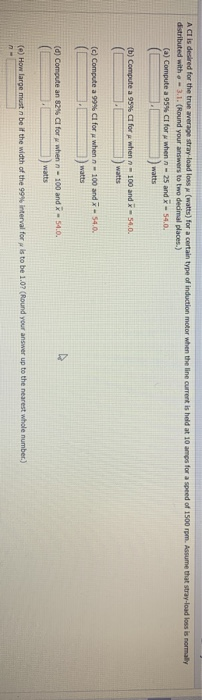A CI is desired for the true average stray.load loss (watts) for a certain type of induction motor when the line current is held at 10 amps for a speed of 1500 rpm. Assume that stray.load loss is normally distributed with a 3.1. (Round your answers to two decimal places.) (a) Compute a 95% CI for when - 25 and X - 54.0. (b) Compute a 95% CI for when - 100 and X - 54.00 watts (c) Compute a 99% CI for when n 100 and X - 54.0. ) watts (d) Compute an 82% Cl for when n - 100 and X - 54.0. ) watts (e) How large must be if the width of the 99% interval for p is to be 1.07(Round your answer up to the nearest whole number)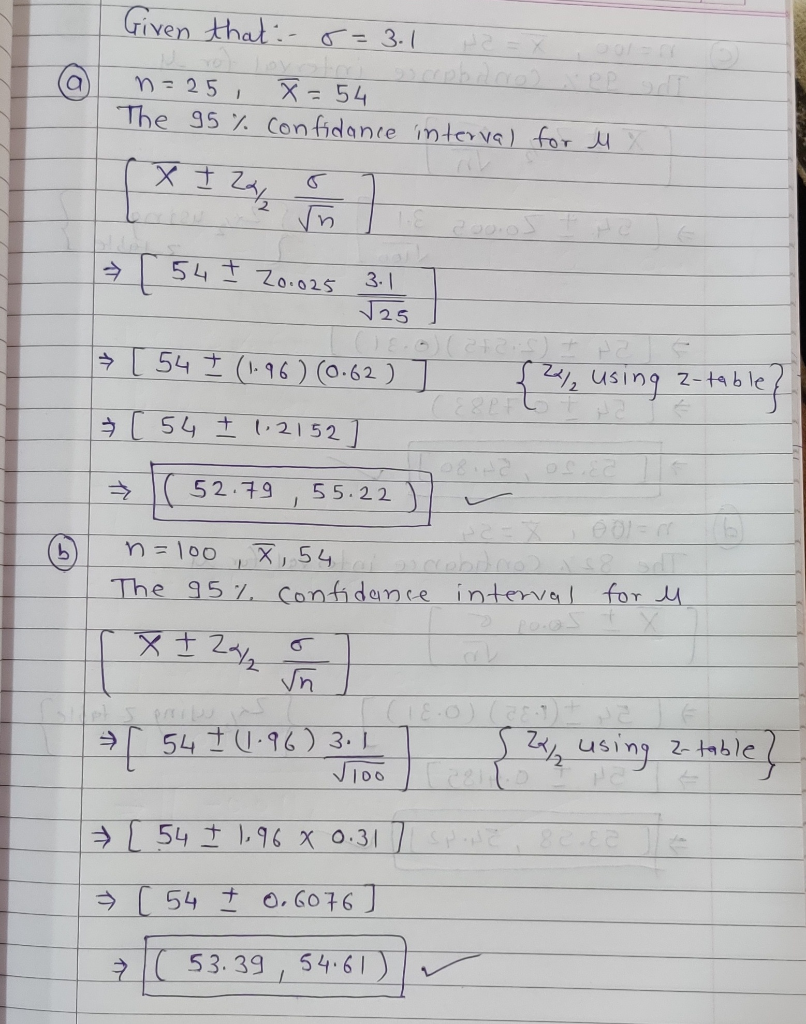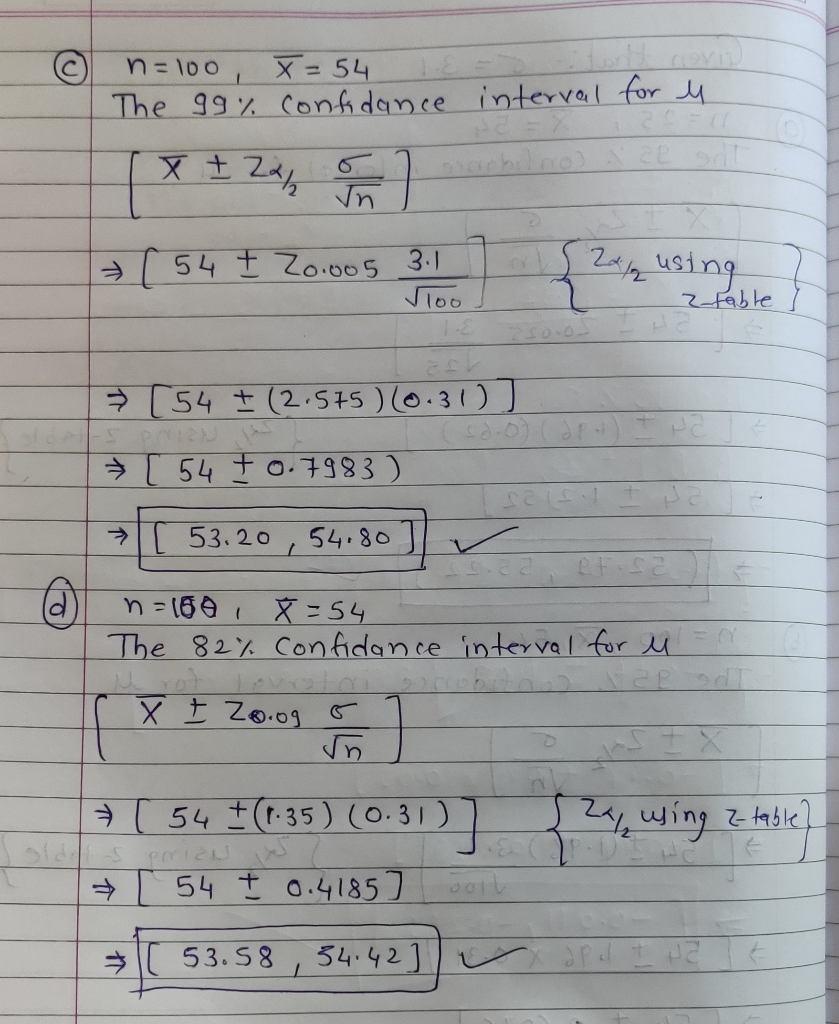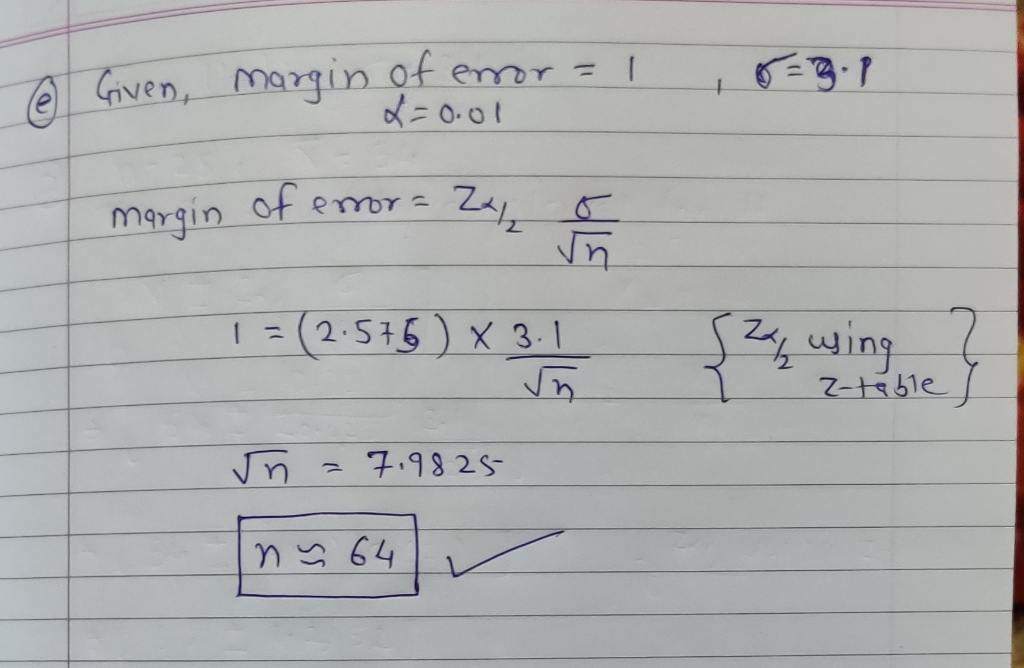#### Earn Coin

Coins can be redeemed for fabulous gifts.

Similar Homework Help Questions
• ### A CI is desired for the true average stray-load loss μ (watts) for a certain type...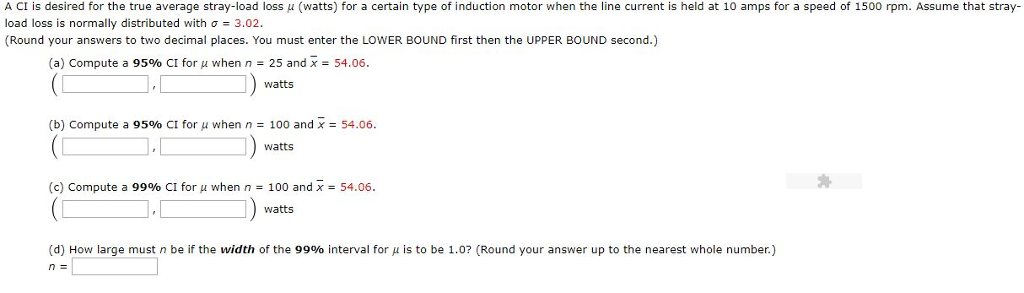A CI is desired for the true average stray-load loss μ (watts) for a certain type of induction motor when the line current is held at 10 amps for a speed of 1500 rpm. Assume that stray load loss is normally distributed with Ơ 3.02. (Round your answers to two decimal places. You must enter the LOWER BOUND first then the UPPER BOUND second.) (a) Compute a 95% CI for μ when n-25 and X-54.06. watts (b) Compute a 95%...

• ### A CI is desired for the true average stray-load loss (watts) for a certain type of...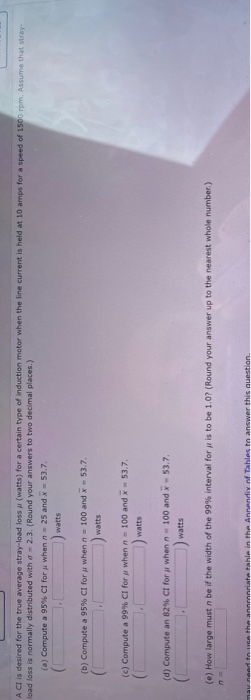A CI is desired for the true average stray-load loss (watts) for a certain type of induction motor when the line current is held at 10 amps for a speed of 1500 rpm. Assume that stray- load loss is normally distributed with a = 2.3. (Round your answers to two decimal places.) (a) Compute a 95% CI for when n25 and x = 53.7. watts (b) Compute a 95% CI for when 100 and 53.7. watts (c) Compute a 99%...

• ### A CI is desired for the true average stray-load loss u (watts) for a certain type...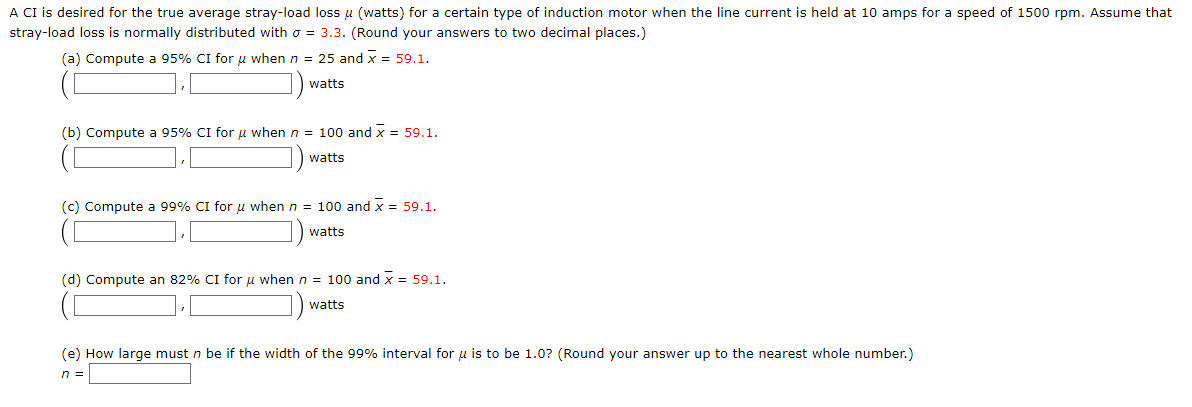A CI is desired for the true average stray-load loss u (watts) for a certain type of induction motor when the line current is held at 10 amps for a speed of 1500 rpm. Assume that stray-load loss is normally distributed with o = 3.3. (Round your answers to two decimal places.) (a) Compute a 95% CI for u when n = 25 and x = 59.1. watts C (b) Compute a 95% CI for u when n = 100...

• ### A CI is desired for the true average stray-load loss u (watts) for a certain type...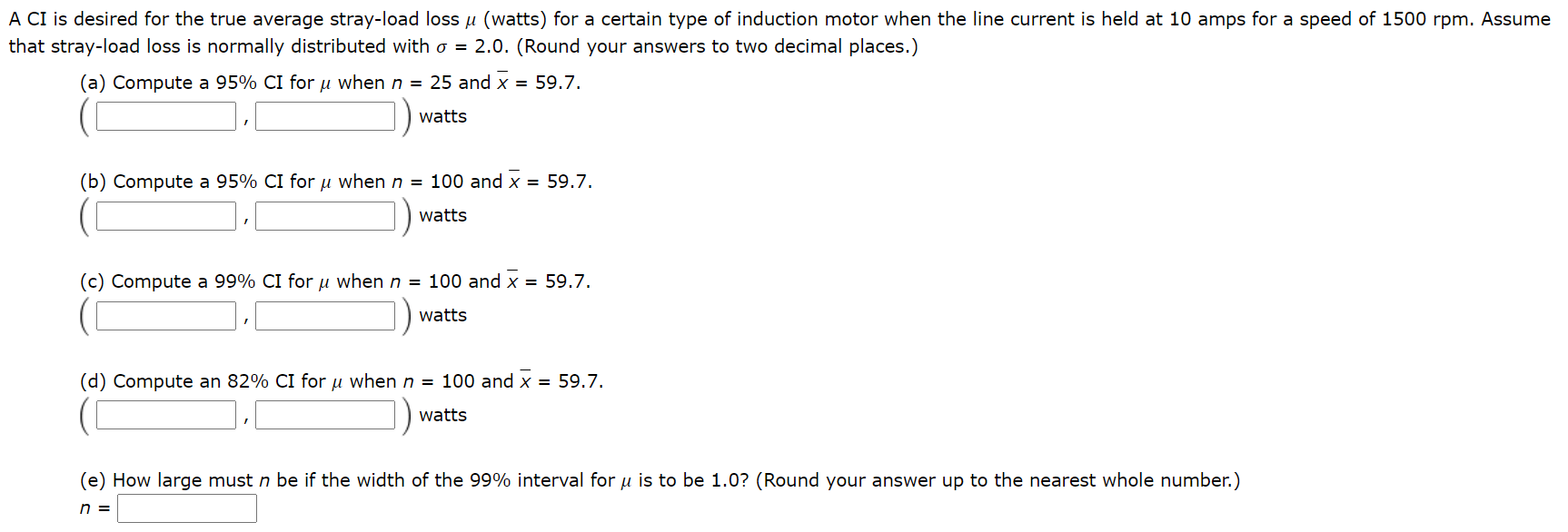A CI is desired for the true average stray-load loss u (watts) for a certain type of induction motor when the line current is held at 10 amps for a speed of 1500 rpm. Assume that stray-load loss is normally distributed with o = 2.0. (Round your answers to two decimal places.) (a) Compute a 95% CI for u when n = 25 and x = 59.7. watts (b) Compute a 95% CI for u when n = 100 and...

• ### A CI is desired for the true average stray-load loss μ (watts) for a certain type...

A CI is desired for the true average stray-load loss μ (watts) for a certain type of induction motor when the line current is held at 10 amps for a speed of 1500 rpm. Assume that stray-load loss is normally distributed with σ = 3.3. (Round your answers to two decimal places.) (a) Compute a 95% CI for μ when n = 25 and x = 57.5.   , watts (b) Compute a 95% CI for μ when n = 100...

• ### A CI is desired for the true average stray-load loss u (watts) for a certain type...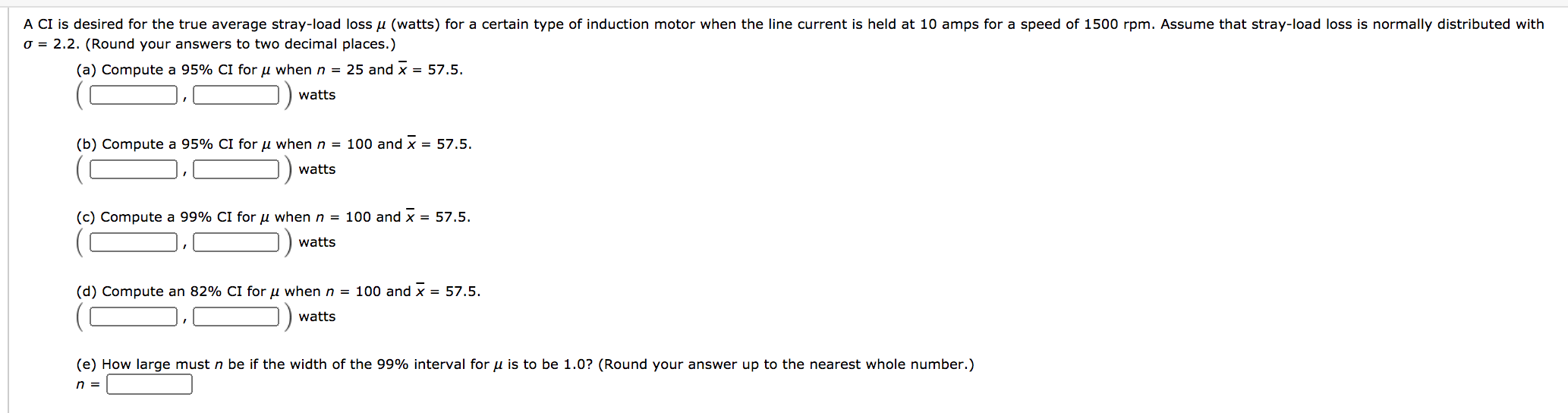A CI is desired for the true average stray-load loss u (watts) for a certain type of induction motor when the line current is held at 10 amps for a speed of 1500 rpm. Assume that stray-load loss is normally distributed with o = 2.2. (Round your answers to two decimal places.) (a) Compute a 95% CI for u when n = 25 and x = 57.5. watts (b) Compute a 95% CI for u when n = 100 and...

• ### A CI is desired for the true average stray-load loss u (watts) for a certain type...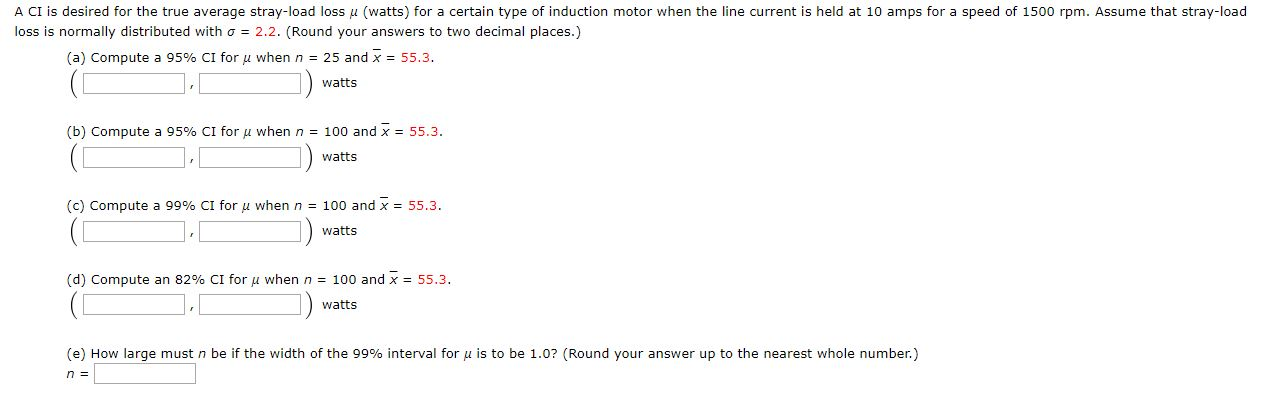A CI is desired for the true average stray-load loss u (watts) for a certain type of induction motor when the line current is held at 10 amps for a speed of 1500 rpm. Assume that stray-load loss is normally distributed with o = 2.2. (Round your answers to two decimal places.) (a) Compute a 95% CI for u when n = 25 and x = 55.3. watts (b) Compute a 95% CI for u when n = 100 and...

• ### A CI is desired for the true average stray-load loss u (watts) for a certain type...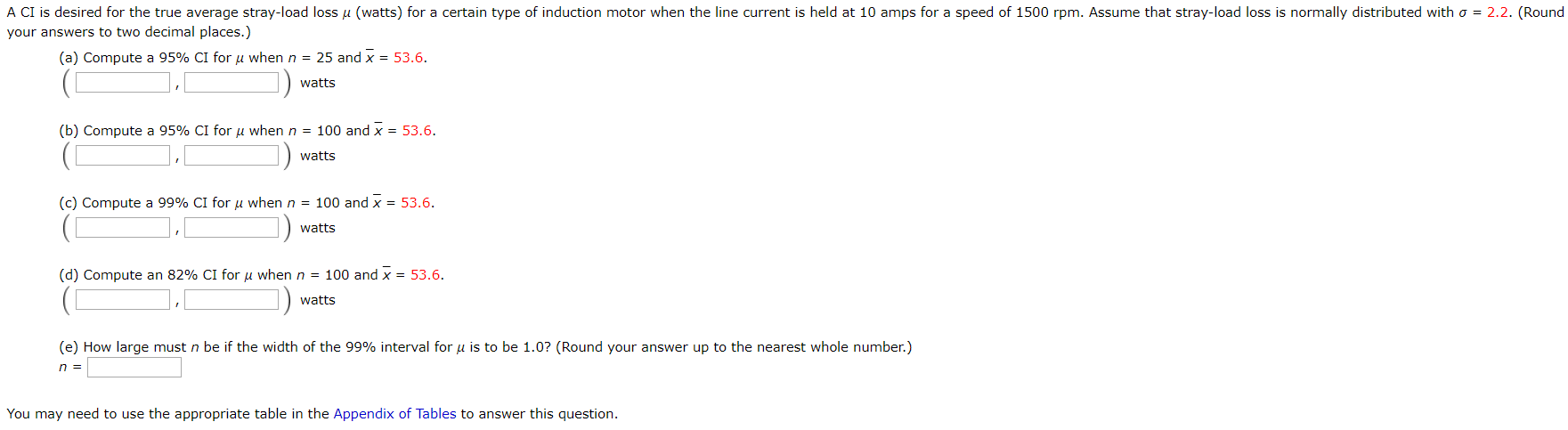A CI is desired for the true average stray-load loss u (watts) for a certain type of induction motor when the line current is held at 10 amps for a speed of 1500 rpm. Assume that stray-load loss is normally distributed with o = 2.2. (Round your answers to two decimal places.) (a) Compute a 95% CI for u when n = 25 and x = 53.6. , watts (b) Compute a 95% CI for u when n = 100...

• ### A Cl is desired for the true average stray-load loss p (watts) for a certain type...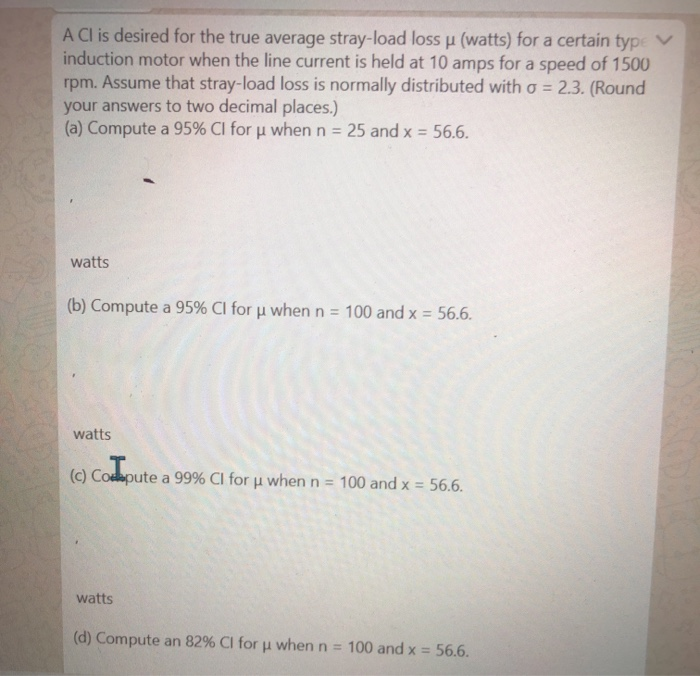A Cl is desired for the true average stray-load loss p (watts) for a certain type induction motor when the line current is held at 10 amps for a speed of 1500 rpm. Assume that stray-load loss is normally distributed with o = 2.3. (Round your answers to two decimal places.) (a) Compute a 95% CI for u when n = 25 and x = 56.6. watts (b) Compute a 95% CI for u when n = 100 and x...

• ### A CI is desired for the true average stray load loss μ watts for a certain...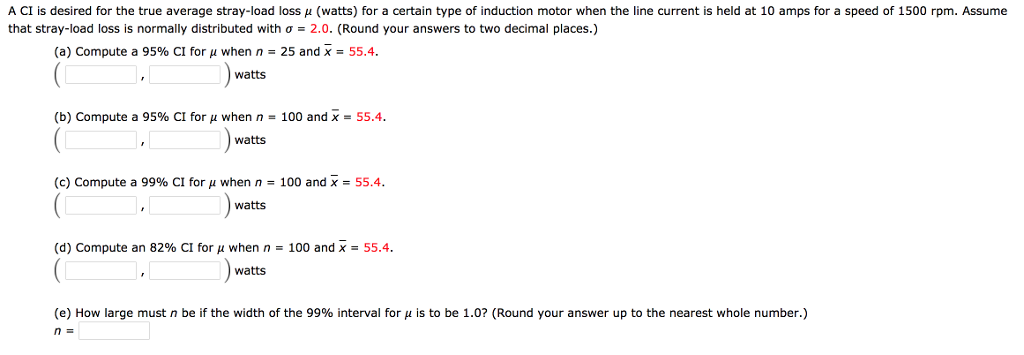A CI is desired for the true average stray load loss μ watts for a certain type of induction motor when the line current is held at 10 am s raspeed of L that stray-load loss is normally distributed with σ = 2.0. (Round your answers to two decimal places.) rpm. Assume (a) Compute a 95% CI for μ when n = 25 and x = 55.4. watts (b) Compute a 95% CI for μ when n = 100 and...

Free Homework App